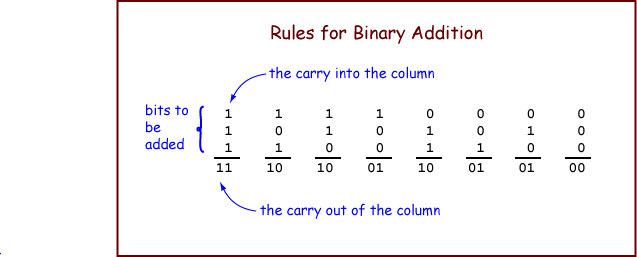In binary:

```0 + 0 = 0
0 + 1 = 1
1 + 0 = 1
1 + 1 = 10
```

The binary addition algorithm is a bit-pattern manipulation procedure that is built into the hardware of (nearly) all computers. All computer scientists and computer engineers know it.Let us start by adding 1-bit integers. The operation is performed on three bits. Arrange the bits into a column. The bit at the top of the column is called the "carry into the column". The operation produces a two-bit result. The left bit of the result is called the "carry out of the column".

To add two 1-bit (representations of) integers: Count the number of ones in a column and write the result in binary. The right bit of the result is placed under the column of bits. The left bit is called the "carry out of the column". The table shows the outcomes with all possible operands.

### QUESTION 2:

```  1             0             1